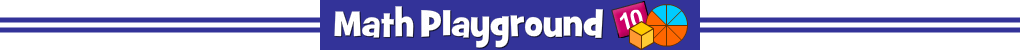Fantastic Fraction and Decimal Games
Game Spotlight: Puppy Chase
Super Starters
Brain Builders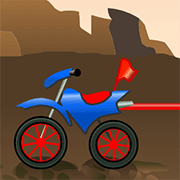###### Compare Fractions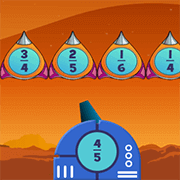###### Galaxy Pals###### Decimal Words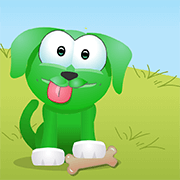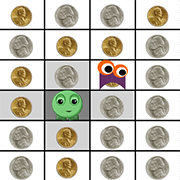###### Zogs and Monsters \$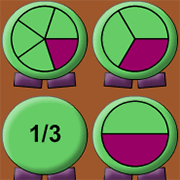###### Triplets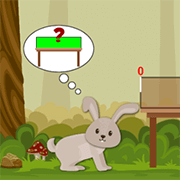###### Playground 2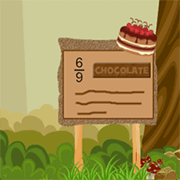###### Equal Fractions 2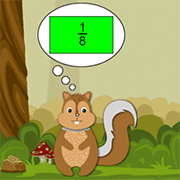###### Equal Fractions 1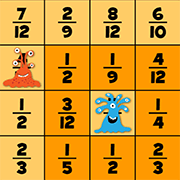###### Monster Stroll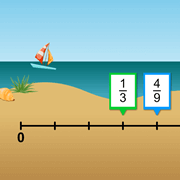###### Number Lines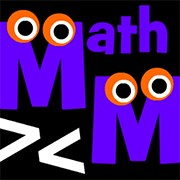###### Math Monster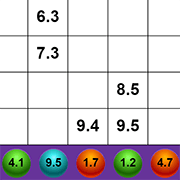###### Treasure Quest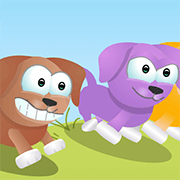###### Convert Fractions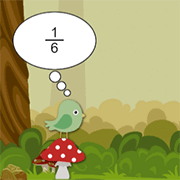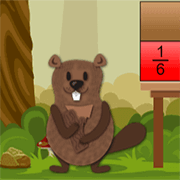###### Playground 3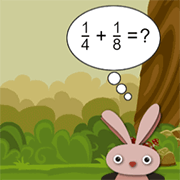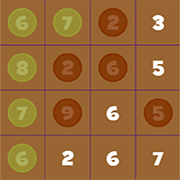###### Math Surpass Fractions
Cranium Challenges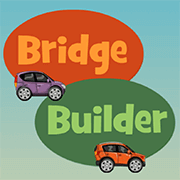###### Bridge Builder Decimals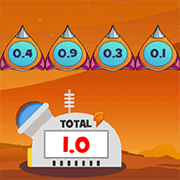###### Galaxy Pals Decimals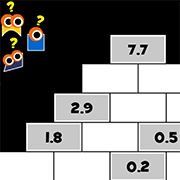###### Decimal Number Puzzles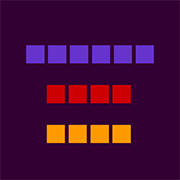###### Mystery Box Fractions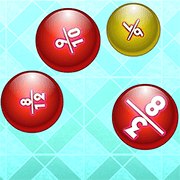###### Ordering Fractions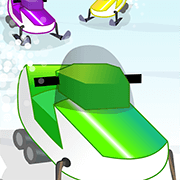###### Multiply Fractions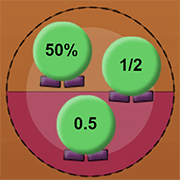###### Decention Jr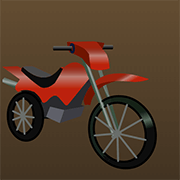###### Equal Ratios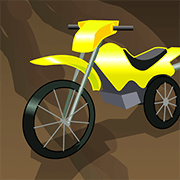###### Proportions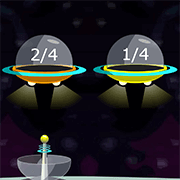###### Equal Ratios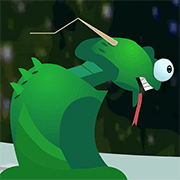###### Ratio Expressions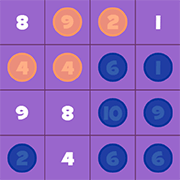###### Math Surpass Percent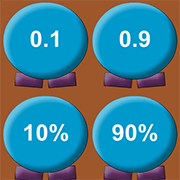###### Decention
Word Problems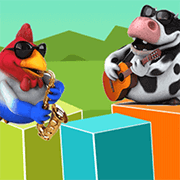###### Thinking Blocks Fractions###### Thinking Blocks Ratios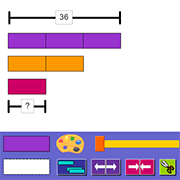###### Modeling Tool
Super Math Puzzles (Subscription Required)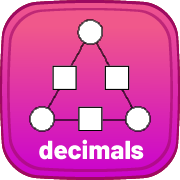###### Triangle Decimals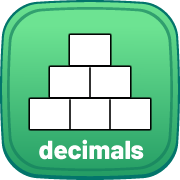###### Pyramid Decimals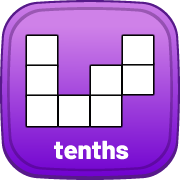###### Decimal Chart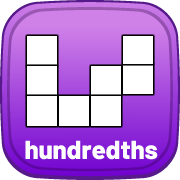###### Decimal Chart Pro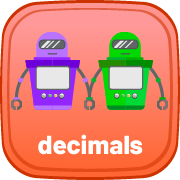###### Function Machine Decimals
Videos - Fractions and Decimals
###### How do you add fractions?How do you reduce fractions?Change fractions into decimalsHow do you divide fractions?Find a fraction of a numberCompare and order fractionsHow do you multiply decimals?How do you divide decimals?Divide decimal by powers of 10
Videos - Percent and Ratios
###### What are equal ratios?Solving ratio word problemsRatiosProportionsHow do you find the sale price?Convert fractions/decimals to percentsCalculating the tipPercentsPercent Change